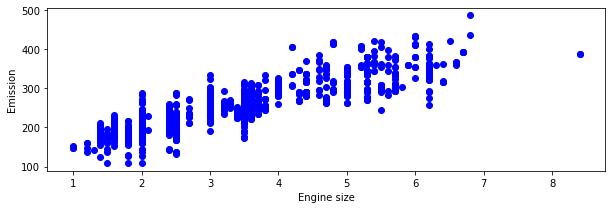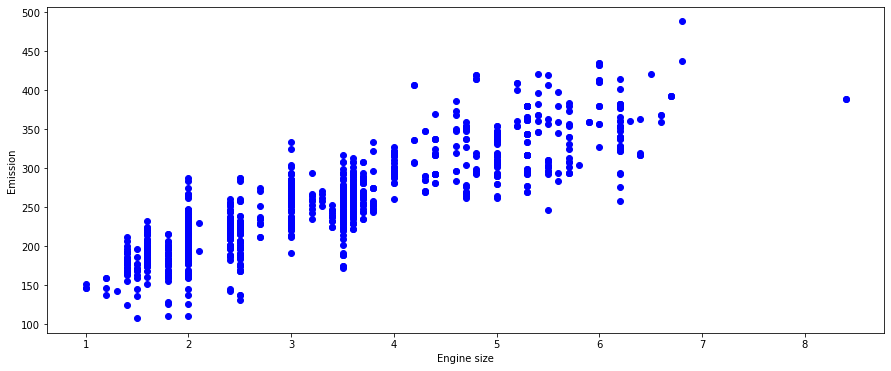0%
Note - Multiple Linear Regression Full Implementation with the dataset EDA and Other Techniques. You will use use the most basic and the Multiple Linear model to predict the car consumption fuel results. Please Download the FuelConsumption.Csv dataset from Kaggle

# Multiple Linear Regression

In this notebook, we learn how to use scikit-learn to implement Multiple linear regression. We download a dataset that is related to fuel consumption and Carbon dioxide emission of cars. Then, we split our data into training and test sets, create a model using training set, Evaluate your model using test set, and finally use model to predict unknown value

### Importing Needed packages¶

In :
import matplotlib.pyplot as plt
import pandas as pd
import pylab as pl
import numpy as np
%matplotlib inline


You will use use the most basic and the Multiple from start to end Linear model to predict the car consumption fuel results. Please Download the FuelConsumption.Csv dataset from Kaggle

## Understanding the Data

### FuelConsumption.csv:¶

We have downloaded a fuel consumption dataset, FuelConsumption.csv, which contains model-specific fuel consumption ratings and estimated carbon dioxide emissions for new light-duty vehicles for retail sale in Canada. Dataset source

• MODELYEAR e.g. 2014
• MAKE e.g. Acura
• MODEL e.g. ILX
• VEHICLE CLASS e.g. SUV
• ENGINE SIZE e.g. 4.7
• CYLINDERS e.g 6
• TRANSMISSION e.g. A6
• FUELTYPE e.g. z
• FUEL CONSUMPTION in CITY(L/100 km) e.g. 9.9
• FUEL CONSUMPTION in HWY (L/100 km) e.g. 8.9
• FUEL CONSUMPTION COMB (L/100 km) e.g. 9.2
• CO2 EMISSIONS (g/km) e.g. 182 --> low --> 0

In :
df = pd.read_csv("FuelConsumption.csv")

# take a look at the dataset
df.info()

<class 'pandas.core.frame.DataFrame'>
RangeIndex: 1067 entries, 0 to 1066
Data columns (total 13 columns):
#   Column                    Non-Null Count  Dtype
---  ------                    --------------  -----
0   MODELYEAR                 1067 non-null   int64
1   MAKE                      1067 non-null   object
2   MODEL                     1067 non-null   object
3   VEHICLECLASS              1067 non-null   object
4   ENGINESIZE                1067 non-null   float64
5   CYLINDERS                 1067 non-null   int64
6   TRANSMISSION              1067 non-null   object
7   FUELTYPE                  1067 non-null   object
8   FUELCONSUMPTION_CITY      1067 non-null   float64
9   FUELCONSUMPTION_HWY       1067 non-null   float64
10  FUELCONSUMPTION_COMB      1067 non-null   float64
11  FUELCONSUMPTION_COMB_MPG  1067 non-null   int64
12  CO2EMISSIONS              1067 non-null   int64
dtypes: float64(4), int64(4), object(5)
memory usage: 108.5+ KB

In :
df.head(5)

Out:
MODELYEAR MAKE MODEL VEHICLECLASS ENGINESIZE CYLINDERS TRANSMISSION FUELTYPE FUELCONSUMPTION_CITY FUELCONSUMPTION_HWY FUELCONSUMPTION_COMB FUELCONSUMPTION_COMB_MPG CO2EMISSIONS
0 2014 ACURA ILX COMPACT 2.0 4 AS5 Z 9.9 6.7 8.5 33 196
1 2014 ACURA ILX COMPACT 2.4 4 M6 Z 11.2 7.7 9.6 29 221
2 2014 ACURA ILX HYBRID COMPACT 1.5 4 AV7 Z 6.0 5.8 5.9 48 136
3 2014 ACURA MDX 4WD SUV - SMALL 3.5 6 AS6 Z 12.7 9.1 11.1 25 255
4 2014 ACURA RDX AWD SUV - SMALL 3.5 6 AS6 Z 12.1 8.7 10.6 27 244

## Lets select some features that we want to use for regression.¶

In :
cdf = df[['ENGINESIZE','CYLINDERS','FUELCONSUMPTION_CITY','FUELCONSUMPTION_HWY','FUELCONSUMPTION_COMB','CO2EMISSIONS']]


## Lets plot Emission values with respect to Engine size:¶

In :
#plt.scatter(cdf.iloc[:,0:1], cdf.iloc[:, 5:6], color = 'blue')
plt.figure(figsize = (10,3))
plt.scatter(cdf.ENGINESIZE, cdf.CO2EMISSIONS,  color='blue')
plt.xlabel("Engine size")
plt.ylabel("Emission")
plt.show()#### Creating train and test dataset¶

Train/Test Split involves splitting the dataset into training and testing sets respectively, which are mutually exclusive. After which, you train with the training set and test with the testing set. This will provide a more accurate evaluation on out-of-sample accuracy because the testing dataset is not part of the dataset that have been used to train the data. It is more realistic for real world problems.

This means that we know the outcome of each data point in this dataset, making it great to test with! And since this data has not been used to train the model, the model has no knowledge of the outcome of these data points. So, in essence, it’s truly an out-of-sample testing.

In :
import random
x = df.loc[: , ['ENGINESIZE','CYLINDERS','FUELCONSUMPTION_COMB']]
y = df.loc[:, ['CO2EMISSIONS']]
print('Independent Variables x shape:' , x.shape , '\n' , 'Dependent Variables y shape:' , y.shape)

#TO USE THIS METHID FIRST SPLIT TO TRAIN AND TEST FIRST AND THEN SPLIT TO X AND Y

#random.seed(2) #SAME NUMBER SO THAT ALL PEOPLE CAN GET THE SAME RESULTS
#msk = np.random.rand(len(df)) < 0.8
#train = cdf[msk]
#test = cdf[~msk]

from sklearn.model_selection import train_test_split
x_train, x_test, y_train, y_test = train_test_split(x , y , train_size = 0.8, test_size = 0.2, random_state = 14)
print(x_train.shape , '\n' , x_test.shape , '\n', y_train.shape , '\n' , y_test.shape)

Independent Variables x shape: (1067, 3)
Dependent Variables y shape: (1067, 1)
(853, 3)
(214, 3)
(853, 1)
(214, 1)


#### Train data distribution¶

In :
plt.figure(figsize = (15,6))
plt.scatter(x.ENGINESIZE, y.CO2EMISSIONS,  color='blue')
plt.xlabel("Engine size")
plt.ylabel("Emission")
plt.show()

#here we can visualize that there is some linear co-realtion that is why we thought of using linear regression## Multiple Regression Model

In reality, there are multiple variables that predict the Co2emission. When more than one independent variable is present, the process is called multiple linear regression. For example, predicting co2emission using FUELCONSUMPTION_COMB, EngineSize and Cylinders of cars. The good thing here is that Multiple linear regression is the extension of simple linear regression model.

In :
from sklearn.linear_model import LinearRegression

regr = LinearRegression()
regr.fit (x_train, y_train)
# The coefficients
print ('Coefficients: ', regr.coef_)
print('Intercepts:', regr.intercept_)

Coefficients:  [[10.46518783  7.80348963  9.41989717]]
Intercepts: [66.59282561]


As mentioned before, Coefficient and Intercept , are the parameters of the fit line. Given that it is a multiple linear regression, with 3 parameters, and knowing that the parameters are the intercept and coefficients of hyperplane, sklearn can estimate them from our data. Scikit-learn uses plain Ordinary Least Squares method to solve this problem.

#### Ordinary Least Squares (OLS)¶

OLS is a method for estimating the unknown parameters in a linear regression model. OLS chooses the parameters of a linear function of a set of explanatory variables by minimizing the sum of the squares of the differences between the target dependent variable and those predicted by the linear function. In other words, it tries to minimizes the sum of squared errors (SSE) or mean squared error (MSE) between the target variable (y) and our predicted output ($\hat{y}$) over all samples in the dataset.

OLS can find the best parameters using of the following methods:

- Solving the model parameters analytically using closed-form equations
- Using an optimization algorithm (Gradient Descent, Stochastic Gradient Descent, Newton’s Method, etc.)

## Prediction

In :
import math
y_hat= regr.predict(x_test)
print(math.sqrt(np.mean(y_test)-np.mean(y_hat)))

1.235646895151453


## Practice

Try to use a multiple linear regression with the same dataset but this time use FUEL CONSUMPTION in CITY and FUEL CONSUMPTION in HWY instead of FUELCONSUMPTION_COMB. Does it result in better accuracy?

## Evaluation¶

In :
#we can directly initialize classes like this too
mse = sklearn.metrics.mean_squared_error(y_test, y_hat)
rmse = math.sqrt(mse)
print('Root Mean Squared Error :',rmse)

from sklearn.metrics import r2_score
#import statsmodels.api as sm

print('r2_score is :', r2_score(y_hat,y_test))

#est = sm.OLS(y, x2)
#est2 = est.fit()
#print(est2.summary())

Root Mean Squared Error : 23.09064357449966

r2_score is : 0.8299022074881567


Due to some reason i can not run this, Please run the above cell. This will give us the p-value and the r2 score too. We can elinimate the values with the p-value below 0.05 and consider the features which have the p-value more than 0.05 which is our significance level. We should run this test till we have all the features with p-value less than 0.05 eliminated.